# KVPY-SX 2019 Physics Paper with Solutions

KVPY-SX is an examination conducted every year for Class 12 students willing to take up a career in science research. KVPY aims at motivating the students by providing scholarships. The examination is conducted by the Department of Technology & Science, Government of India. KVPY-SX 2019 Physics paper with solutions are provided here. Solving the previous years’ question papers helps the students to get a better understanding of the pattern of the question paper.

### KVPY SX 2019 - Physics

Question 1: In a muonic atom, a muon of mass of 200 times of that of electron and same charge is bound to the proton. The wavelengths of its Balmer series are in the range of

1. (a) X-rays
2. (b) infrared
3. (c) γ rays
4. (d) microwave

Solution:

As we know that the, energy of an orbit is given by,

$E=\frac{-me^{4}Z^{2}}{8\epsilon _{0}^{2}h^{2}n^{2}}$

m = mass of electron

z = number protons/ Atomic no.

h = planks constant

n = no. of series / integer value

Since, Mass = 200 times of that of electron i.e. mμ = 200me

Energy directly proportional to mass

⇒ Energy increases by 200 times.

$E=\frac{hc}{\lambda }\Rightarrow E\propto \frac{1}{\lambda }\Rightarrow \frac{E_{1}}{E_{2}}=\frac{\lambda _{2}}{\lambda _{1}}$

Let E1 = E So, E2 = 200 E

$\frac{E_{1}}{E_{2}}=\frac{\lambda_{2} }{\lambda _{1}}\Rightarrow \frac{\lambda_{2} }{\lambda _{1}}=\frac{E}{200E}$

$\Rightarrow \lambda _{2}=\frac{1}{200}\lambda _{1}$

Where λ2 = wavelength for corresponding Balmer series.

λ1 = wavelength for corresponding hydrogen atom.

⇒ Wavelength will decrease by 200 times.

So, the wavelength λ for corresponding Balmer series will be 1/200 times that of hydrogen atom, so it will be in range of X-rays.

Hence the correct option is (a).

Question 2: We consider the Thomson model of the hydrogen atom in which the proton charge is distributed uniformly over a spherical volume of radius 0.25 Angstrom. Applying the Bohr condition in this model the ground state energy (in eV) of the electron will be close to

1. (a) -13.6/4
2. (b) –13.6
3. (c) -13.6/2
4. (d) –2 × 13.6

Solution:

For electron orbiting around a proton

By Bohr’s condition

RH = 0.529 Å [RH = radius of 1st Bohr orbit]

Given, r = 0.25 Å

Which is less than 0.529 Å

And we can say that if electron is orbiting a proton or a positive sphere having charge equal to proton then its Kinetic energy & Potential energy will be – 13.6 eV

∴ K.E. = P.E. of electron

∴TE = –13.6 eV

So, total energy is same as Bohr’s model = –13.6 eV.

Question 3: A spherical rigid ball is released from rest and starts rolling down an inclined plane from height h = 7 m, as shown in the figure. It hits a block at rest on the horizontal plane (assume elastic collision). If the mass of both the ball and the block is m and the ball is rolling without sliding, then the speed of the block after collision is close to: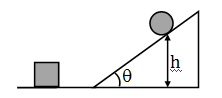1. (a) 6 m/s
2. (b) 8 m/s
3. (c) 10 m/s
4. (d) 12 m/s

Solution:

Using energy conservation for ball

Kinetic energy + rotational energy = Potential energy.

$\frac{1}{2}mv^{2}+\frac{1}{2}I\omega ^{2}=mgh$

m = mass of ball

v = linear velocity of ball

$\omega$= angular velocity of ball

h = height of ball from ground

I = moment of inertia of ball (sphere)

Ball is a sphere and moment of inertia of sphere = $=\left [ \frac{2}{5}mR^{2} \right ]$

$\frac{1}{2}mV^{2}+\frac{1}{2}\left [ \frac{2}{5}mR^{2} \right ]\frac{v^{2}}{R^{2}}=mgh$

{Since Relation between linear velocity and angular velocity is $v=\omega R\Rightarrow \omega =\frac{v}{R}$}

$\frac{1}{2}v^{2}+\frac{1}{5}v^{2}=gh\Rightarrow \left [ \frac{5+2}{10} \right ]v^{2}=gh$

$\Rightarrow v=\sqrt{\frac{10gh}{7}}$

Therefore, $\Rightarrow v=\sqrt{\frac{10\times 10\times 7}{7}}$ (since g = acceleration due to gravity = 10m/s2)

Therefore, v = 10 m/s

As the collision is elastic and mass of block & ball are same, so after collision it will transfer whole velocity to the block.

Therefore, Vblock = 10 m/s

Question 4: A girl drops an apple from the window of a train which is moving on a straight track with speed increasing with a constant rate. The trajectory of the falling apple as seen by the girl is

1. (a) Parabolic and in the direction of the moving train
2. (b) Parabolic and opposite to the direction of the moving train
3. (c) An inclined straight line pointing in the direction of the moving train
4. (d) An inclined straight line pointing opposite to the direction of the moving train

Solution:

Let the acceleration of train is ‘a’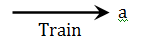In the frame of train, for apple when it is dropped; a pseudo force will act on it due to which it will get an acceleration same as that of train but in opposite direction to that of train; also, acceleration due to gravity will act downwards.

$\Rightarrow$For apple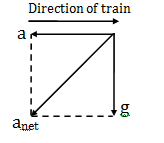$a_{net}=\sqrt{a^{2}+g^{2}}$

The trajectory of the falling apple as seen by the girl is an incline straight line pointing opposite to the direction of the moving train, as relative initial velocity is zero.

Question 5: A train is moving slowly at 2 m/s next to a railway platform. A man, 1.5 m tall, alights from the train such that his feet are fixed on the ground. Taking him to be a rigid body, the instantaneous angular velocity (in rad/sec) is:

1. (a) 1.5
2. (b) 2.0
3. (c) 2.5
4. (d) 3.0

Solution:

Man is considered a rigid body.

Applying conservation of angular momentum about feet just before and after landing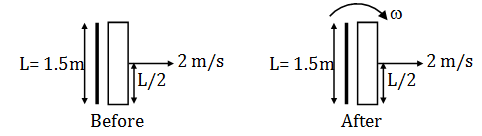$mv\frac{L}{2}=I\omega$

Where I = moment of inertia and here moment of inertia about a fixed point or on end is ML2/3 (considering like uniform thin rod)

$mv\frac{L}{2}=\frac{mL^{2}}{3}\times \omega$

$\Rightarrow \omega =\frac{3v}{2L}$

Substituting the values L = 1.5 m, v = 2 m/s

$\Rightarrow \omega =\frac{3\times 2}{2\times 1.5}=2 rad/sec$

Question 6: A point mass M moving with a certain velocity collides with a stationary point mass M/2. The collision is elastic and in one dimension. Let the ratio of the final velocity of M and M/2 be x. The value of x is:

1. (a) 2
2. (b) 3
3. (c) 1/2
4. (d) 1/4

Solution:

Let before collision velocity of mass M is u1 and mass M/2 is 0 because it is stationary.

Before collision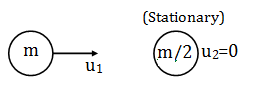After collision let the velocity of mass M is v1 and velocity of mass M/2 is v2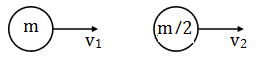By linear momentum conservation

pi = pf

mu1 = mv1 + mv2/2

u1 = v1 + v2/2 … (1)

Now, as collision is elastic so,

Coefficient of restitution e = Velocity of separation (after collision)/Velocity of approach (before collision)

e =( v2-v1)/u1 = 1

u1 = v2 – v1 … (2)

By equation (1) &equation (2)

v2 = (4/3) u1 & v1 = u1/3

So, $\frac{v_{1}}{v_{2}}=\frac{u_{1}/3}{4u_{1}/3}=\frac{1}{4}$

Question 7: A particle of mass 2/3 kg with velocity v = –15 m/s at t = –2 s is acted upon by a force f = k – βt2. Here k = 8 N and β = 2 N/s2. The motion is one dimensional. Then the speed at which the particle acceleration is zero again, is:

1. (a) 1 m/s
2. (b) 16 m/s
3. (c) 17 m/s
4. (d) 32 m/s

Solution:

From the definition of force F (Force) = mass × acceleration

F = ma {since ,acceleration a =dv/dt }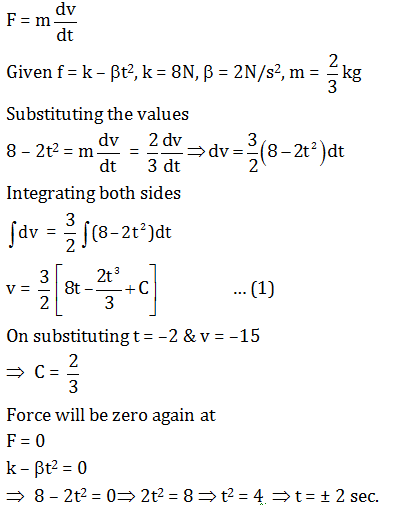Time cannot be negative therefore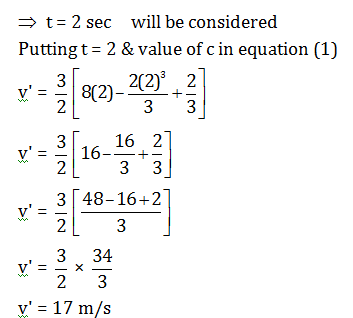Question 8: A certain stellar body has radius 50 Rs and temperature 2Ts and is at a distance of 2 × 1010 A.U. from the Earth. Here A.U. refers to the earth sun distance and Rs and Ts refer to the sun's radius and temperature respectively. Take both star and sun to be ideal black bodies. The ratio of the power received on earth from the stellar body as compared to that received from the sun is close to:

1. (a) 4 × 10–20
2. (b) 2 × 10–6
3. (c) 10–8
4. (d) 10–16

Solution:

From kirchoff’s law power of stellar body i.e. Energy per unit time.

PBody = e $\sigma$AT4

P = Power

A = surface area

T = temperature

$\sigma$= Stefan Boltzmann const.

E = emissivity of block body (e = 1)

Temperature of staller body = 2Ts

Where Ts = Temperature of sun Now, Substituting the values.

PBody = 1. $\sigma$.(4 $\pi$ (50RS)2)(2TS)4

{As the bodies are ideal black bodies, so emissivity e = 1}

PBody = 502 × 24 × Psun …………. (1)

Since,Intensity is given by Energy per unit area per unit time i.e. $I=\frac{E}{At}=\frac{P_{body}}{A}$

Intensity at earth due to body = $\frac{P_{body}}{4\pi (2\times 10^{4}AV)^{2}}$ …………. (2)

From equation (1) & (2)

$\frac{50^{2}\times 2^{4}\times P_{sum}}{4\times 10^{20}\times (4\pi AV)^{2}}$

$\frac{50^{2}\times 2^{4}}{4\times 10^{20}}\times I_{sun}$

$\Rightarrow I_{Body}=10^{-6}I_{sun}$

Question 9: As shown in the schematic diagram below, a rod of uniform cross-sectional area A and length l is carrying a constant current i through it and voltage across the rod is measured using an ideal voltmeter. The rod is stretched by the application of a force F. Which of the following graphs would show the variation in the voltage across the rod as function of the strain, ε, when the strain is small. Neglect Joule heating.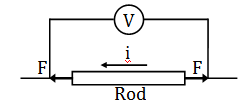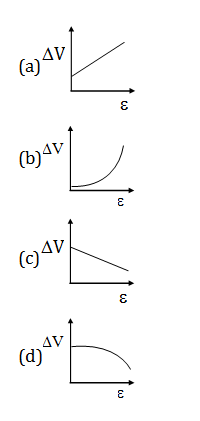Solution:

From ohm’s law

V = iR

V = voltage

i = current

R = Resistance

i->constant

V = i(Ro + ΔR) ………….. (1)

When current i flows through the conducting wire the resistance is given by

R = ρl/A …………. (2)

Where, ρ = resistivity of wire (Rod), A = Area of cross section, l = length of wire (Rod).

Let change in resistance is ΔR,

Let change in length is Δl,

Let change in Area is ΔA,

Therefore, Equation (2) becomes.

$\frac{\Delta R}{R}=\rho \left [ \frac{\Delta l}{l}-\frac{\Delta A}{A} \right ]$

$\frac{\Delta A}{R}=- \left [ \frac{\Delta l}{l} \right ]$ and ρ is constant because there is no joule heating

$\Delta R=\rho R\left ( \frac{2\Delta l}{l} \right )$

= $=2\rho R\varepsilon$

Substituting it in equation (1) we get

$V=i(R+2\rho R\varepsilon )$

Since, V will increase linearly with strain from an initial value.

So, graph will look like.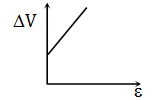Question 10: Two identical coherent sound sources R and S with frequency f are 5m apart. An observer standing equidistant from the sources and at a perpendicular distance of 12 m from the line RS hears maximum sound intensity when he moves parallel to RS. The sound intensity varies and is minimum when he comes directly in front of one of the two sources. Then a possible value of f is close to (the speed of sound is 330 m/s)

1. (a) 495 Hz
2. (b) 275 Hz
3. (c) 660 Hz
4. (d) 330 Hz

Solution: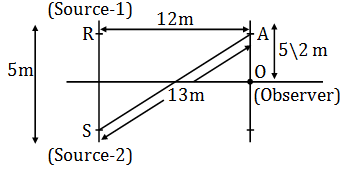Here when observer hears minimum sound intensity the path difference will be odd multiple of $\lambda /2$.

AS – AR = (2n + 1) $\lambda /2$= (2n + 1)(330/2f)

13 – 12 = (2n + 1)(330/2f)

f = 165(2n + 1)

To find a possible of f

Put n = 1

f = 495 Hz.

Question 11: A photon falls through a height of 1 km through the earth's gravitational field. To calculate the change in its frequency, take its mass to be hν/c2. The fractional change in frequency ν is close to:

1. (a) 10–20
2. (b) 10–17
3. (c) 10–13
4. (d) 10–10

Solution:

Total energy of photon will be,

Total energy of photon = Kinetic energy + Potential energy of photon

hv' = hv + mgh

Therefore, h(v' – v) = mgh ………………. (1)

Given that; mass (m) = hv/c2

Equation (1) becomes

Therefore, h(v' – v) =hv/c2 ×gh

To find the fractional change in frequency, above equation divided by v

$\frac{v-v^{'}}{v}=\frac{gh}{c^{2}}$

So, substituting the values of acceleration due to gravity

G = 9.8 m/s2, Height (h) = 1 km = 1 × 103 m, speed of light c = (3 × 108) m/s.

$\frac{v-v^{'}}{v}=\frac{9.8\times 1\times 10^{3}}{(3\times 10^{8})^{2}}$ = 1.1 × 10–13

Question 12: 0.02 moles of an ideal diatomic gas with initial temperature 20°C is compressed from 1500 cm3 to 500 cm3. The thermodynamic process is such that PV2 = β where β is a constant. Then the value of β is close to: (The gas constant, R = 8.31 J/K/mol)

1. (a) 7.5 × 10–2 Pa.m6
2. (b) 1.5 × 102 Pa.m6
3. (c) 3 × 10–2 Pa.m6
4. (d) 2.2 × 101 Pa.m6

Solution:

Given that, PV2 = β ……(1)

(Number of moles) n = 0.02, f = 5, T1 = 20°C

V1 = 1500 × 10–6, V2 = 500 × 10–6

By ideal gas equation

PV = nRT

$\Rightarrow P=\frac{nRT}{V}$

(n = number of moles, R = gas constant, V = volume, T = Temperature, P = pressure)

Substituting the value of P in equation (1)

$\frac{nRT}{V}.V^{2}=\beta$

$\Rightarrow$ nRTV = β

Substituting the value of n, R, T and V

$\Rightarrow$ 0.02 × 8.31 × (273 + 20) × 1500 × 10–6 = β

$\Rightarrow$ β = 0.073

$\Rightarrow$ β= 7.5 × 10–2 Pa.m6

Question 13: A heater supplying constant power P watts is switched on at time t = 0 minutes to raise the temperature of a liquid kept in a calorimeter of negligible heat capacity. A student records the temperature of the liquid T(t) at equal time intervals. A graph is plotted with T(t) on the y-axis versus t on the x-axis. Assume that there is no heat loss to the surrounding heating. Then,

1. (a) the graph is a straight line parallel to the time axis
2. (b) the heat capacity of the liquid is inversely proportional to the slope of the graph
3. (c) if some heat were lost at a constant rate to the surroundings during heating, the graph would be a straight line but with a larger slope
4. (d) the internal energy of the liquid increases quadratically with time

Solution:

From the definition of specific heat s = Q /mdT ⇒msdT …………. (1)

Where Q = amount of heat, m = mass of the substance, dT = change in temperature.

Let power supplied by the heater is ‘P’ and dT be the change in temperature.

Therefore, P = ms(dT/dt)

Therefore, dT =P/ms × dt

Integrating both sides

$\int_{0}^{T}dT=\int_{0}^{T}\frac{P}{ms}\times dt$

$\Rightarrow T(t)=\frac{P}{ms}T+\theta _{0}$

Comparing it with straight line equation y = mx + C

(Slope) = m =P/ms , C = θ0

θ0 $\rightarrow$ temperature at t = 0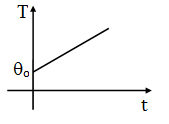Question 14: Unpolarized red light is incident on the surface of a lake at incident angle θR. An observer seeing the light reflected from the water surface through a polarizer notices that on rotating the polarizer, the intensity of light drops to zero at a certain orientation. The red light is replaced by unpolarized blue light. The observer sees the same with reflected blue light at incident angle θB, then:

1. (a)θBR< 45°
2. (b)θB = θR
3. (c)θBR> 45°
4. (d)θBR> 45°

Solution:

By Cauchy’s theorem, we know that

µ = µo + A/ λ2

µ 0, A are coefficients that can be determined for a material by fitting the equation to measured refractive indices at known wavelengths. The coefficients are usually quoted for λ as the vacuum wavelength in micrometers.

µred< µblue

As the reflected light is polarized, so incidence angle must be equal to Brewster’s angle.

by Brewster law

µ = tan iB, µ = refractive index, iB = angle of incidence of blue light.

$\Rightarrow$ iB = tan–1(µ)

$\Rightarrow$ θRB

Question 15: A neutral spherical copper particle has a radius of 10 nm (1 nm = 10–9 m). It gets charged by applying the voltage slowly adding one electron at a time. Then the graph of the total charge on the particle vs the applied voltage would look like: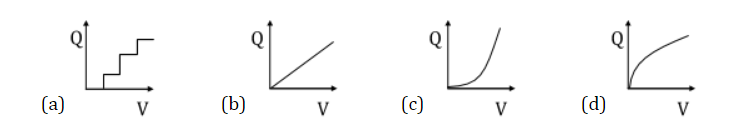Solution:

For spherical surface, potential

V = kQ/r

K = Constant (9 × 109 N–m2/C2)

Q = Magnitude of charge

r = Distance of the charge

Since charge is increasing in discrete manner, so the potential will remain same till the time charge is same on sphere.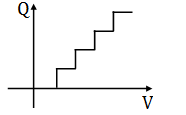Question 16: A charge +q is distributed over a thin ring of radius r with line charge density λ = θsin2θ/(πr). Note that the ring is in the x-y plane and θ is the angle made by with the x-axis. The work done by the electric force in displacing a point charge +Q from the centre of the ring to infinity is:

1. (a) Equal to qQ/2πε0r
2. (b) Equal to qQ/4πε0r
3. (c) Equal to zero only if the path is a straight line perpendicular to the plane of the ring
4. (d) Equal to qQ/8πε0r

Solution:

Given radius of the ring = r, $\lambda =\frac{sin^{2}\theta }{\pi r}$

From the definition of linear charge density $\lambda =\frac{q}{l}$ , here l= r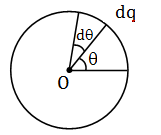Let dθ be the small angle made by small charge element dq so, the potential at the Centre of the ring i.e. $O=\int \frac{k.dq}{r}$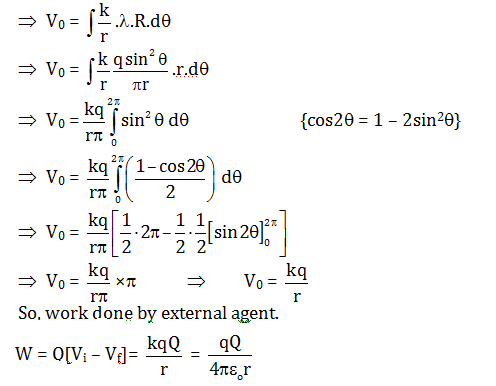Question 17: Originally the radioactive beta decay was thought as a decay of a nucleus with the emission of electrons only (Case I). However, in addition to the electron, another (nearly) massless and electrically neutral particle is also emitted (Case II). Based on the figure below, which of the following is correct: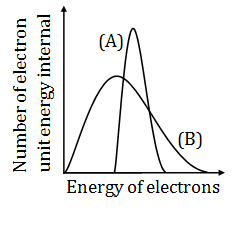1. (a)(A) in both cases I and II
2. (b)(A) in case I and (B) in case II
3. (c)(A) in case II and (B) in case I
4. (d)(B) in both cases I and II

Solution:

In case-I energy of all the electrons will be same as no neutrino or anti-neutrino are coming out but in case-II as neutrino or anti neutrino are coming out so energy of electron will be different.

Question 18: One gram mole of an ideal gas A with the ratio of constant pressure and constant volume specific heats, γA = 5/3 is mixed with n gram-moles of another ideal gas B with γB = 7/5. If the γ for the mixture is 19/13 what will be the value of n?

1. (a) 0.75
2. (b) 2
3. (c) 1
4. (d) 3

Solution:

During the mixing process internal energy remains conserved

By energy conservation

$\Rightarrow \frac{f_{1}}{2}n_{1}RT+ \frac{f_{2}}{2}n_{2}RT=\frac{f_{mix}}{2}(n_{1}+n_{2})RT$

Where f1 = degree of freedom for ideal gas A,

T = temperature

R = gas constant

f2 = degree of freedom for ideal gas B,

n1 = Number of moles of ideal gas A,

n2 = Number of moles of ideal gas B,

fmix = degree of freedom of mixture of gases A & B

$f_{mix}=\frac{f_{1}n_{1}+f_{2}n_{2}}{n_{1}+n_{2}}$

As, $\gamma =1+\frac{2}{f}$ [γ= Ratio of constant pressure and constant volume specific heats}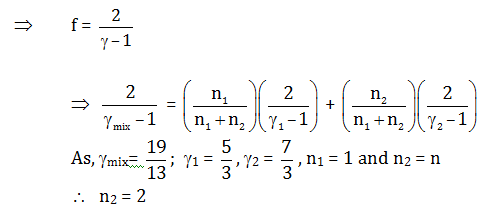Question 19: How will the voltage (V) between the two plates of a parallel plate capacitor depend on the distance (D) between the plates, if the charge on the capacitor remains the same?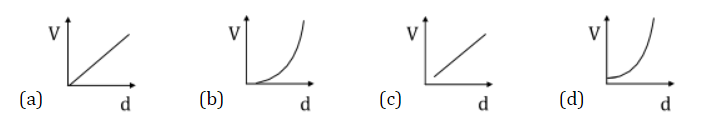Solution:

The charge Q on the plates is proportional to the potential difference V across the two plates

i.e, Q ∝V

⇒ Q = CV ……………. (1)

Where C = Capacitance

We know that as, $C=\frac{\varepsilon _{0}A}{d}\times V$……………... (2)

$\varepsilon _{0}$ = Permittivity of free space

A = Area of plate

D = distance between the plates.

So, from equation (1) & (2)

$\Rightarrow Q =[\varepsilon _{0}A/d]\times V$

$\Rightarrow V =\frac{Qd}{\varepsilon _{0}A}$

Therefore, $\Rightarrow V \propto d$

But as d cannot be zero.

Therefore, Graph in option (c) is correct.

Question 20: Three large identical plates are kept close and parallel to each other. The outer two plates are maintained at temperatures T and 2T, respectively. The temperature of the middle plate in steady state will be close to:

1. (a) 1.1 T
2. (b) 1.3 T
3. (c) 1.7 T
4. (d) 1.9 T

Solution: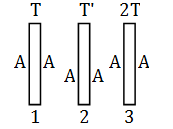A $\rightarrow$ Area of cross section

Let the temperature of middle plate be ‘T’ and the area of all the plates is same i.e, A.

Let us assume that all the plates are perfectly black bodies.

From Stefan Boltzmann law

Rate of Heat loss = Rate of heat gain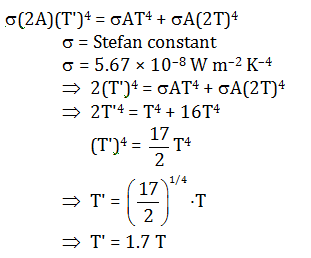Question 21: A metal rod of cross-sectional area 10–4 m2 is hanging in a chamber kept at 20°C with a weight attached to its free end. The coefficient of thermal expansion of the rod is 2.5 × 10–6 K–1 and its Young's modulus is 4 × 1012 N/m2. When the temperature of the chamber is lowered to T then a weight of 5000 N needs to be attached to the rod so that its length is unchanged. Then T is

1. (a) 15°C
2. (b) 12°C
3. (c) 5°C
4. (d) 0°C

Solution:

Given (area) A = 10–4 m2

θ1 = 20°C, θ2 = T, α= 2.5 × 10–6 K–1, Y = 4 × 1012 N/m2

Since, we know that coefficient of linear expansion

α= change in length/original length x rise in temperature

$\alpha =\frac{\Delta l}{l\times \Delta \theta }$ … (1)

And Young’s modulus Y =Fl/A .Δl , where F = force, A = Area

Substituting the values

$4\times 10^{12}=\frac{5000\times l}{10^{-4}\times \Delta l}$

$\Rightarrow \frac{\Delta l}{l}=\frac{5000}{4\times 10^{12}\times 10^{-4}}=1250 \times 10^{-8}$ … (2)

Now from equation (1) & (2)

$\Rightarrow \Delta \theta =\frac{\Delta l}{l\times \alpha }=\frac{1250\times 10^{-8}}{2.5\times 10^{-6}}=500\times 10^{-2}=5$

20 – T=5

$\Rightarrow T=15^{0}C$

Question 22: A short solenoid (length l and radius r, with n turns per unit length) lies well inside and on the axis of a very long, coaxial solenoid (length L, radius R and N turns per unit length, with R > r). Current I flow in the short solenoid. Choose the correct statement.

1. (a) There is uniform magnetic field µ0nI in the long solenoid.
2. (b) Mutual inductance of the solenoids is πµ0r2nNl.
3. (c) Flux through outer solenoid due to current I in the inner solenoid is proportional to the ratio R/r.
4. (d) Mutual inductance of the solenoids is πµ0rRnNlL(rR)1/2.

Solution:

The magnetic flux linked with long solenoid of radius R due to short solenoid of radius r having current I is,

Φ = MI … (1)

I = current

Where M = mutual inductance

The magnetic field due to the short solenoid,

B = µonlI

Φ = B.A

B = magnetic field

A = area

Φ = µonlI(πr2) … (2)

From eq.(1) and (2), we get

M = πµo r2nl

For N number of turns

M = πµo r2Nnl

Question 23: Consider the wall of a dam to be straight with height H and length L. It holds a lake of water of height h(h < H) on one side. Let the density of water be ρw. Denote the torque about the axis along the bottom length of the wall by τ1. Denote also a similar torque due to the water up to height h/2 and wall length L/2 by τ2. Then τ12 (ignore atmospheric pressure) is

1. (a) 2
2. (b) 4
3. (c) 8
4. (d) 16

Solution: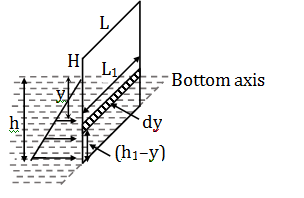Pressure at any height h:

P = Po + ρ gh

P = ρgh {Since given ignore atmospheric pressure}

P ∝h, p0 = atmosphere pressure

Force on elemental area dA:

dF = Py.dA

dF =ρwgydA

dF = ρwgyL1dy

Torque about bottom length when height of water is h1& length of dam is L1 and y = height of water: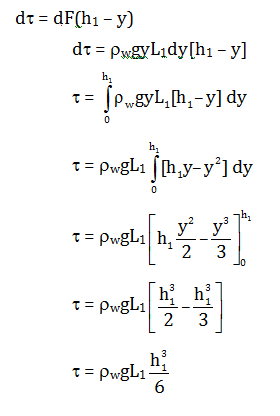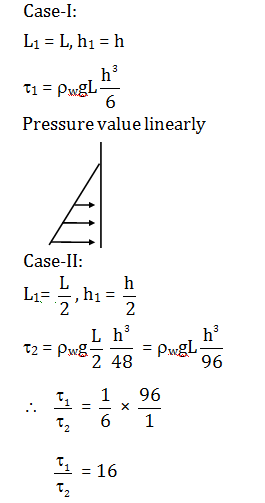Question 24: Two containers C1 and C2 of volumes V and 4V respectively hold the same ideal gas and are connected by a thin horizontal tube of negligible volume with a valve which is initially closed. The initial pressures of the gas in C1 and C2 are P and 5P, respectively. Heat baths are employed to maintain the temperatures in the containers at 300 K and 400 K respectively. The valve is now opened. Select the correct statement:

1. (a) The gas will flow from the hot container to the cold one and the process is reversible.
2. (b) The gas will flow from one container to the other till the number of moles in two containers are equal.
3. (c) A long time after the valve is opened, the pressure in both the containers will be 3P.
4. (d) A long time after the valve is opened, number of moles of gas in the hot container will be thrice that of the cold one.

Solution:

When valve is opened pressure will be same in both the containers after a long time.

So, P1 = P2

Since, From ideal gas equation PV = nRT⇒P = nRT/V

$\frac{n_{1}RT_{1}}{V_{1}}=\frac{n_{2}RT_{2}}{V_{2}}$

n1 = number of moles in container C1

n2 = number of moles in container C2

V1 = V = volume of gas in container C1

V2 = 4V = volume of gas in container C2

T1 = 300 K = temperature of gas in container C1

T2 = 400 K = temperature of gas in container C2

So,

$\frac{n_{1}}{n_{2}}=\frac{T_{2}V_{1}}{T_{1}V_{2}}$

$\frac{n_{1}}{n_{2}}=\frac{400\times V}{300\times 4V}$

$\frac{n_{1}}{n_{2}}=\frac{1}{3}$

Question 25: Four electrons, each of mass me are in a one dimensional box of size L. Assume that the electrons are non-interacting, obey the Pauli exclusion principle and are described by standing de Broglie waves confined within the box. Define α = h2/8meL2 and U0 to be the ground state energy. Then

1. (a) The energy of the highest occupied state is 16α
2. (b) U0 = 30α
3. (c) The total energy of the first excited state is U0 + 9α
4. (d) The total energy of the second excited state is U0 + 8α

Solution:

Electrons are described by standing de Broglie waves confined within the box.

So length will be L = n λ/2 … (1)

Since, De Broglie wavelength λ = h/p … (2)

From equation (1) & (2)

P = hn/2L

Since, Energy $E =\frac{P^{2}}{2m_{e}}=\frac{n^{2}h^{2}}{4L^{2}.2m_{e}}=\frac{n^{2}h^{2}}{8L^{2}m_{e}}$

Given $\alpha =\frac{h^{2}}{8m_{e}L^{2}}$

∴ E = n2α,

For ground state E = α = u0

For first excited state E = 22α = 4u0

= u0 + 3α

For second excited state E = 32α = 9α

= u0 + 8α

Question 26: A rope of length L and uniform linear density is hanging from the ceiling. A transverse wave pulse, generated close to the free end of the rope, travels upwards through the rope. Select the correct option.

1. (a) The speed of the pulse decreases as it moves up
2. (b) The time taken by the pulse to travel the length of the rope is proportional to $\sqrt{L}$
3. (c) The tension will be constant along the length of the rope
4. (d) The speed of the pulse will be constant along the length of the rope.

Solution: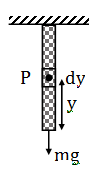Considering the small element of rope dy which is at height y from the bottom or free end of the rope.

So, mass of the total length is = m

Mass of the 1 length is =m/L

Mass of the y length is = (m/L)y … (1)

Mass per unit µ = m/L

eq. (1) becomes µy

Tension in the rope at point P will be T = µyg

As we know that speed of transverse wave in rope is given by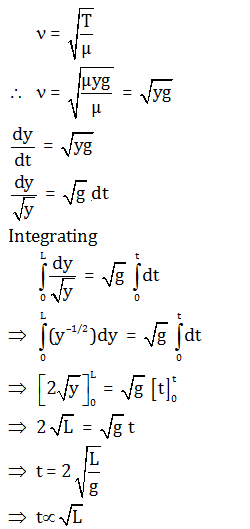Question 27: A circuit consists of a coil with inductance L and an uncharged capacitor of capacitance C. The coil is in a constant uniform magnetic field such that the flux through the coil is Φ. At time t = 0, the magnetic field is abruptly switched off. Let ω0 =$\frac{1}{\sqrt{LC}}$ and ignore the resistance of the circuit. Then,

1. (a) Current in the circuit is I(t) = (Φ/L) cosω0t
2. (b) Magnitude of the charge on the capacitor is |Q(t)| = 2Cω0|sinω0t|
3. (c) Initial current in the circuit is infinite
4. (d) Initial charge on the capacitor is Cω0 Φ

Solution:

From kirchoff’s voltage law

$-\frac{q}{C}=L\frac{di}{dt}$

q = charge

C = capacitance

L = inductance

di/dt= rate of change of current

φ = fuse

Antiquely Lio – 0 = φ

⇒io = φ/L

Since, I = dq/dt

So, from equation (1)

$-\frac{q}{LC}=\frac{d}{dt}\left ( \frac{dq}{dt} \right )$

$-\frac{q}{LC}=\frac{d^{2}q}{dt^{2}}$

⇒ q = q0sinω0t

i = dq/dt = q0ω0cosω0t

i = (φ /L)cosω0t

Question 28: Consider the configuration of a stationary water tank of cross section area A0, and a small bucket as shown in figure below: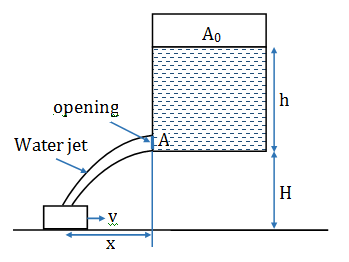What should be the speed, v, of the bucket so that the water leaking out of a hole of cross-section area A (as shown) from the water tank does not fall outside the bucket?

Take h = 5 m, H = 5 m, g = 10 m/s2, A = 5 cm2 and A0 = 500 cm2.

1. (a) 1 m/s
2. (b) 0.5 m/s
3. (c) 0.1 m/s
4. (d) 0.05 m/s

Solution: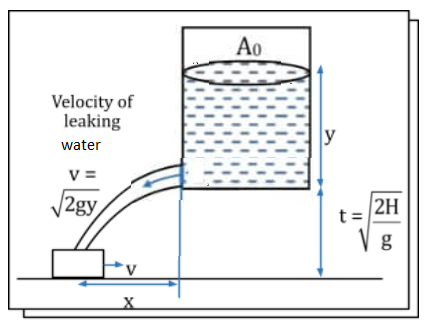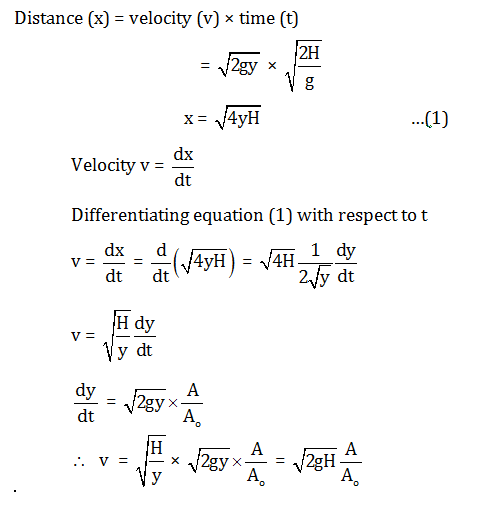Substituting the values

$v=\sqrt{2\times 10\times 5}\times \frac{5}{100}$

$v=\sqrt{100}\times \frac{1}{100}$

$v=\frac{10}{100}=0.1 m/s$

Question 29: The circuit below is used to heat water kept in a bucket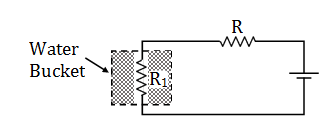Assuming heat loss only by Newton's law of cooling, the variation in the temperature of the water in the bucket as a function of time is depicted by: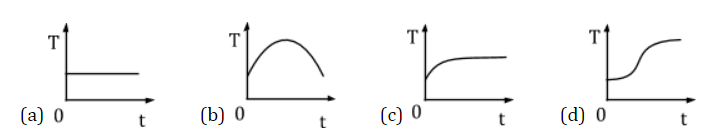Solution:

From Newton’s law of cooling and Joule’s heating effect.

Rate of heat gained by water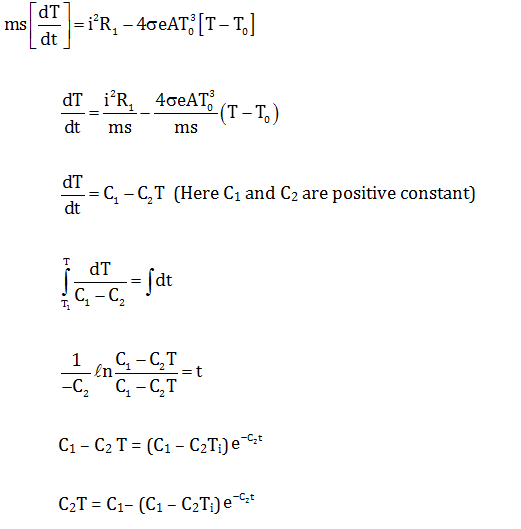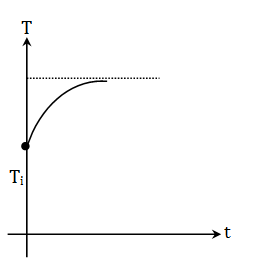Question 30: A bubble of radius R in water of density ρ is expanding uniformly at speed ν. Given that water is incompressible, the kinetic energy of water being pushed is

1. (a) Zero
2. (b) 2πρR3ν2
3. (c) 2πρR3ν2/3
4. (d) 4πρR3ν2/3

Solution: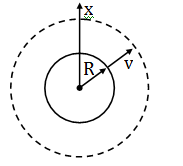As bubble increases its volume, due to surface tension work is to be done, velocity flux will be same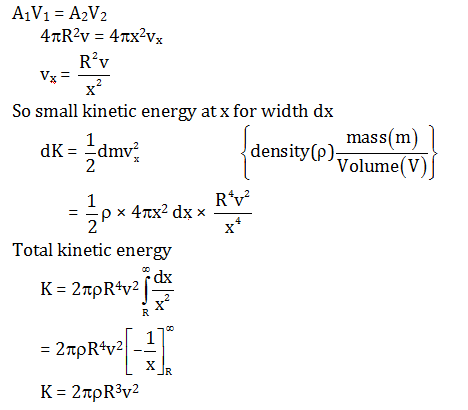### KVPY-SX 2019 Physics Paper with Solutions